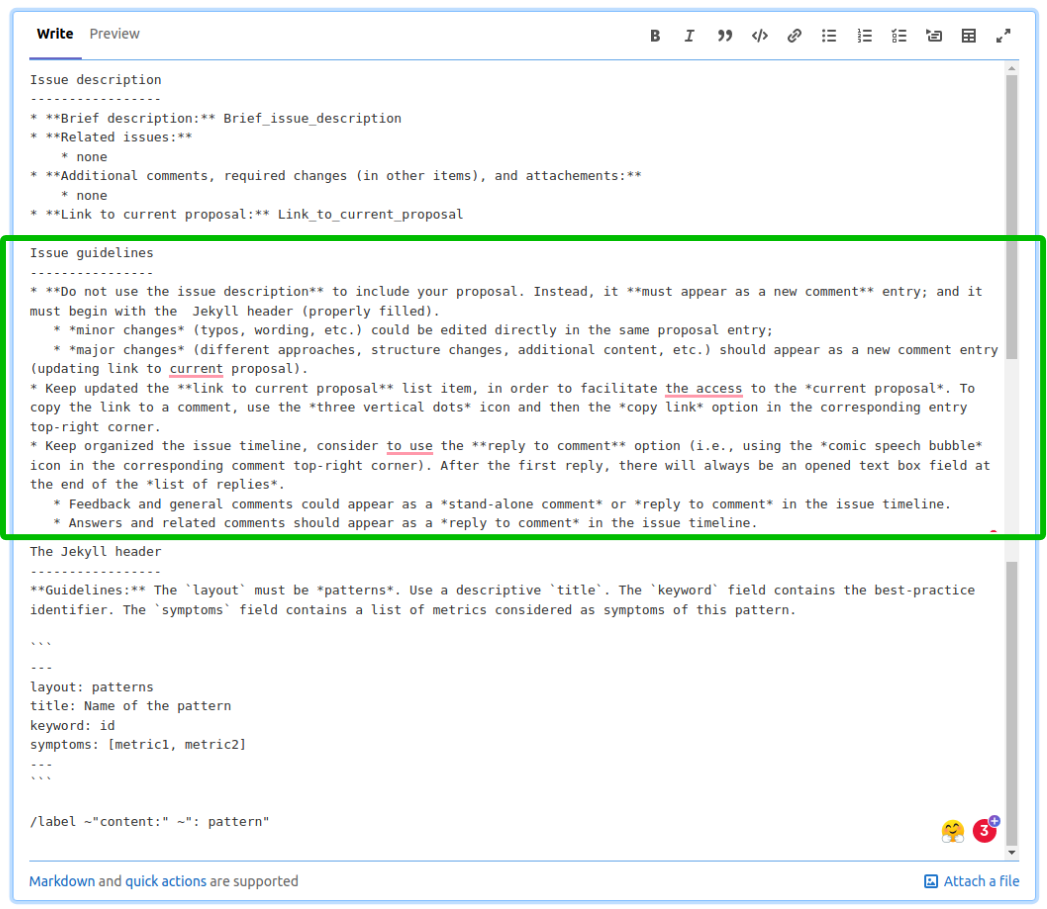# Template of a pattern

Usual symptom(s):
• IPC Scaling: The IPC Scaling (IPCS) compares IPC to the reference case. (more...)
• Load Balance Efficiency: The Load Balance Efficiency (LBE) is computed as the ratio between Average Useful Computation Time (across all processes) and the Maximum Useful Computation time (also across all processes). (more...)

This paragraph should be the first content after the Jekyll header (excerpt). It should be a small paragraph introducing the pattern. This is because when we are listing all patterns in the co-design website, we list the title and the excerpt for each pattern. Thus, here should be placed the highlights of the pattern. In the following sections of the content, we can then provide a detailed description of the pattern.

As the OpenMP is the most commonly used library providing shared-memory parallelism, we present a silver code using the OpenMP constructs. A programmer aims to accelerate the for loop containing a simple operation by utilizing cores available within a single node. A general form of threaded for-loop with the large_number of iterations distributed chunk-wise among num_threads threads with particular chunks being of the size of chunk_size reads as follows.

This is a math formula: $$A \mathbf{v} = \lambda B \mathbf{v}$$

This is an external link: POP websiteThis is a code section:

// Template of a C code with OpenMP clauses
#pragma omp for schedule( dynamic, chunk_size )
for(int i=0; i < large_number; i++){
// simple operation with no dependency
do_simple_operation();
}